Function Repository Resource:

# SinusoidPlotQuiz

Generate a quiz to assess understanding of the graphs of sine and cosine functions

Contributed by: Wolfram|Alpha Math Team
 ResourceFunction["SinusoidPlotQuiz"][] returns an interactive assessment on graphing sinusoids with given attributes.

## Details

ResourceFunction["SinusoidPlotQuiz"][] randomly picks three numbers for the amplitude, period and midline of a sinusoid. The user is asked to plot a sinusoid that exhibits the given parameters.
The interface returned by ResourceFunction["SinusoidPlotQuiz"] includes the following buttons:
 Check answer automatically grades input with feedback Reset problem resets the points to their starting locations Next problem randomly generates a new problem and internally saves the previous problem history Print report prints a description of the problems that have been attempted so far, all guesses made and whether the last guess on each problem was correct
Styling and interface controls may be found under the "Display options" opener.
ResourceFunction["SinusoidPlotQuiz"] accepts the option "Start". The value for "Start" can be either "Parent" or "Random" (the default). When "Random" is used, the initial sinusoid plotted has arbitrarily chosen parameters, and when "Parent" is used, each problem begins with the untranslated graph of Sin[x] or Cos[x].

## Examples

### Basic Examples (1)

Generate an interface to quiz yourself on plotting a sinusoid based on a description of its attributes:

 In:=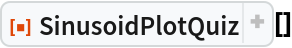Out=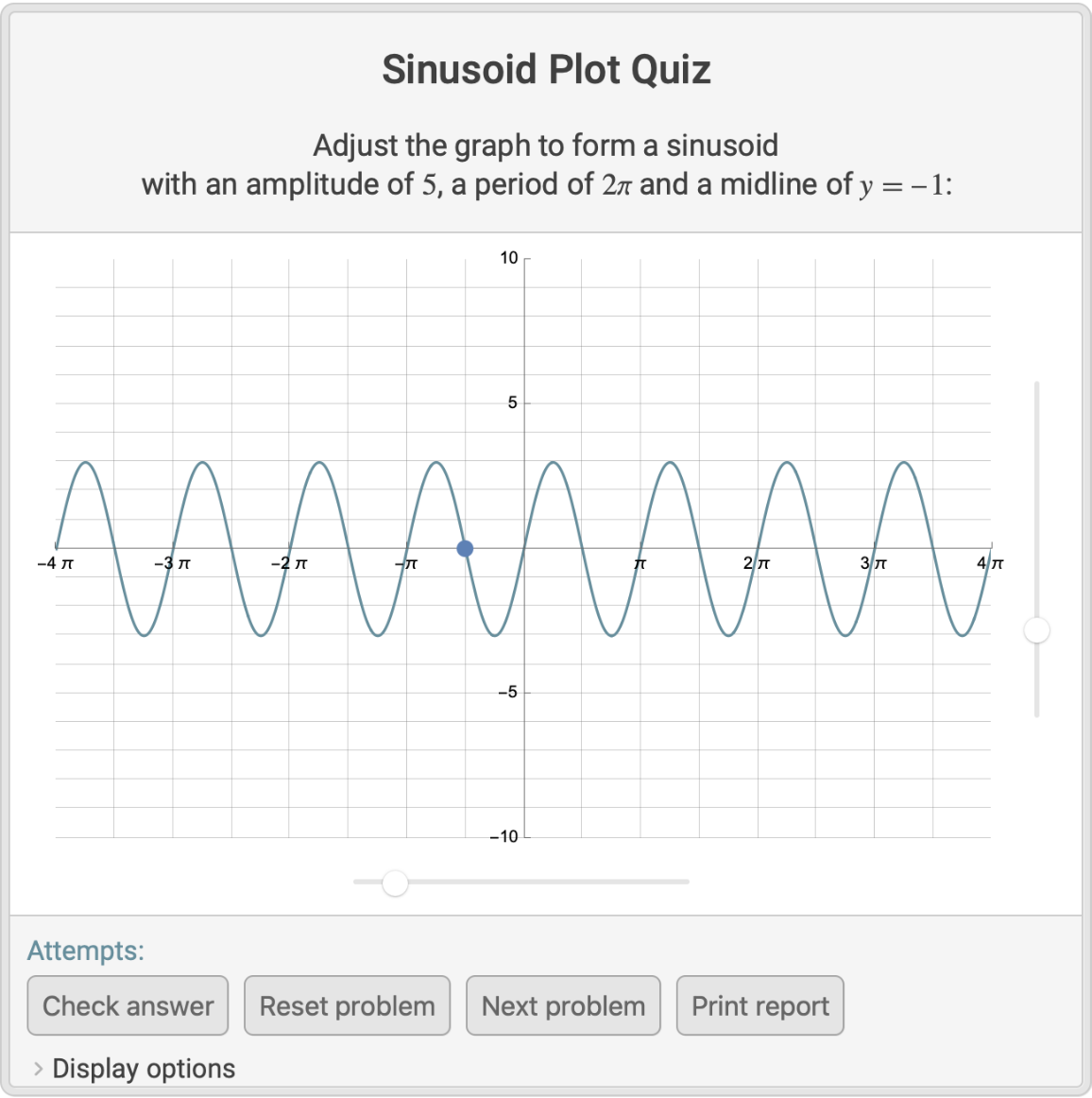### Scope (5)

When the curve demonstrates the approximate amplitude, period and midline described in the prompt, clicking the Check answer button marks the attempt as correct with a green check mark:

 In:=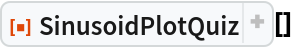Out=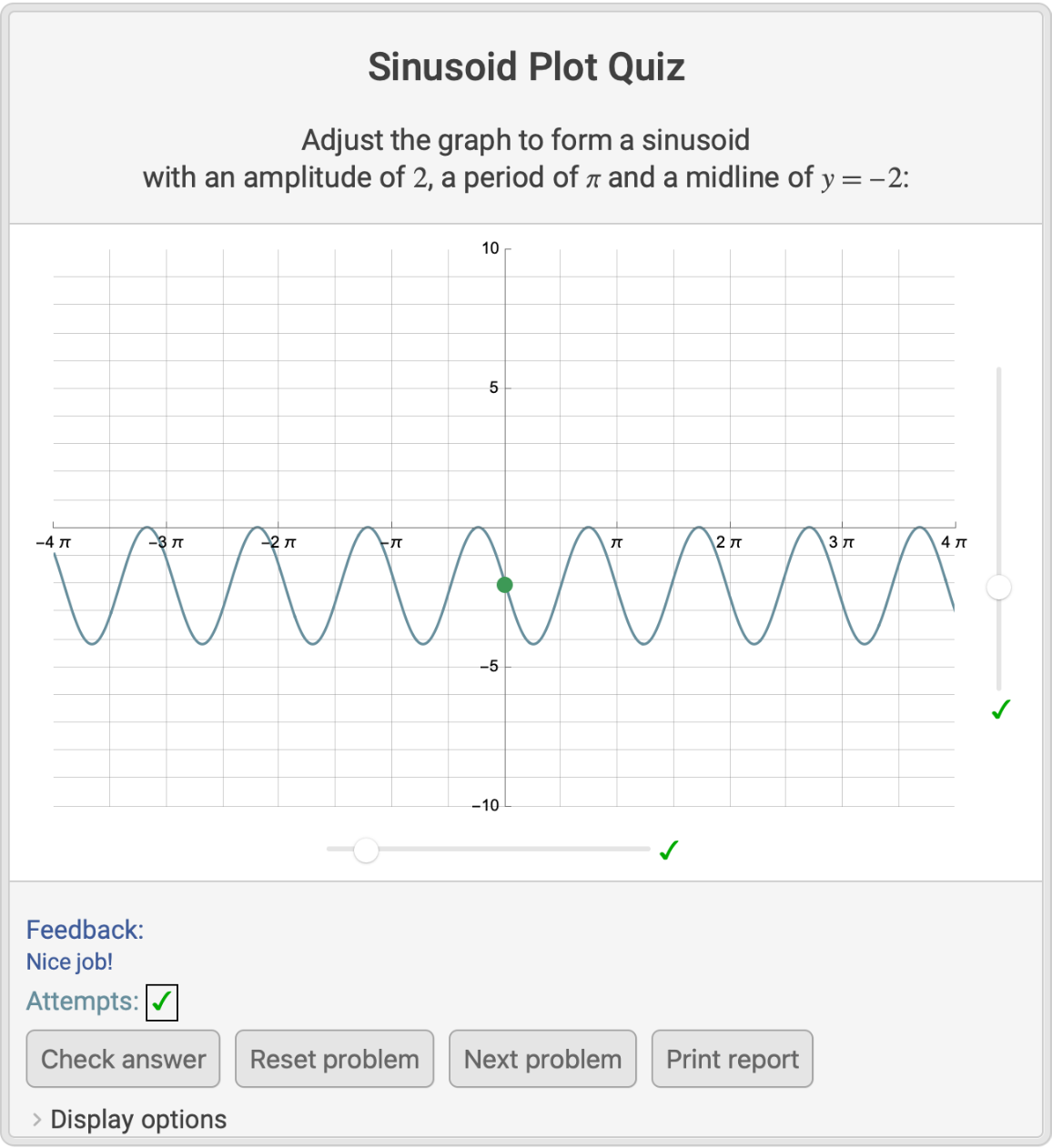If an attempt does not approximately meet the specified criteria, the Check answer button marks the attempt with a red x, and the student receives feedback to guide their next attempt:

 In:=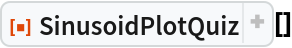Out=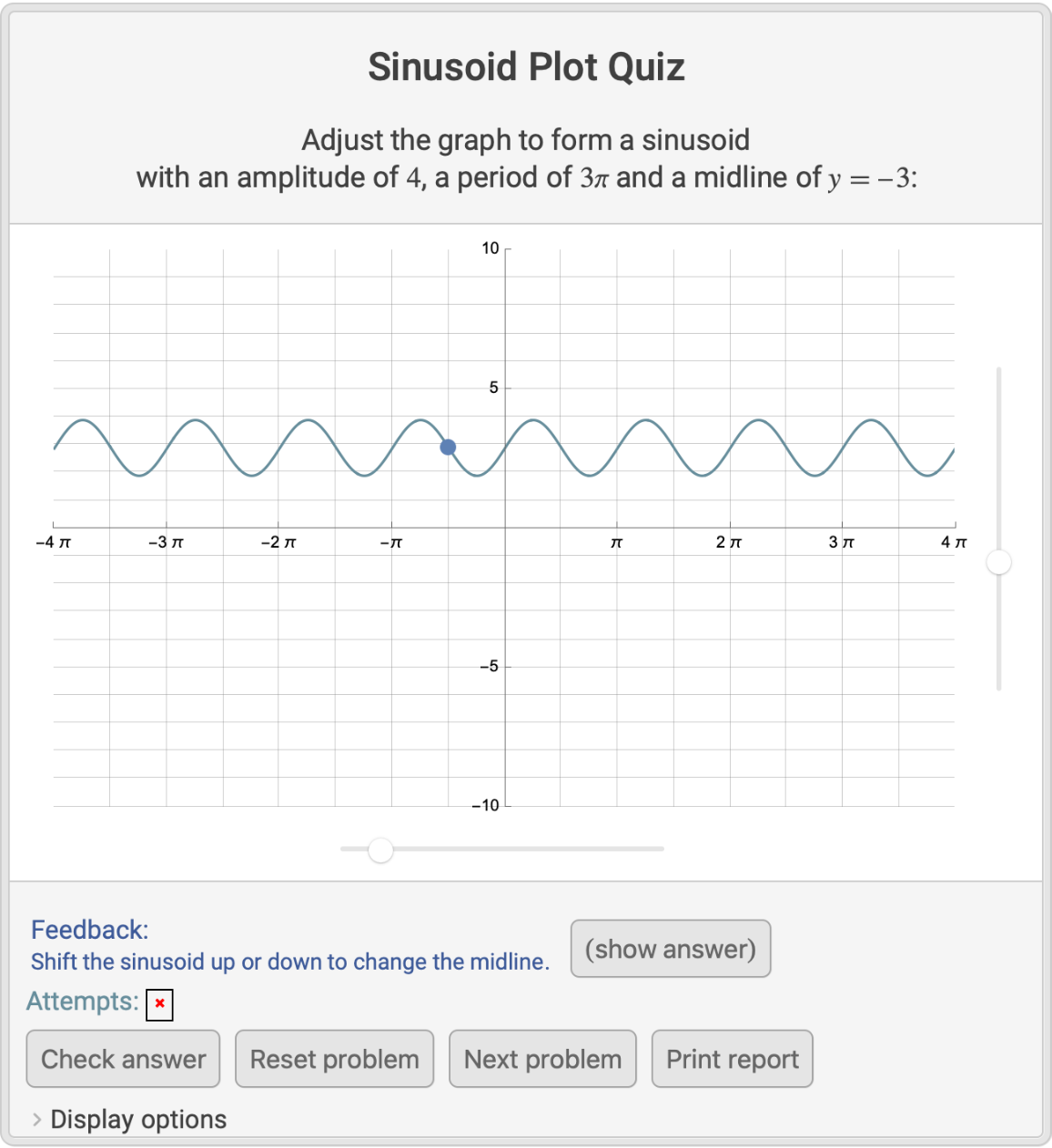Clicking the (show answer) button prints a correct answer and prohibits students from submitting any further answers to the current problem:

 In:=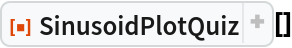Out=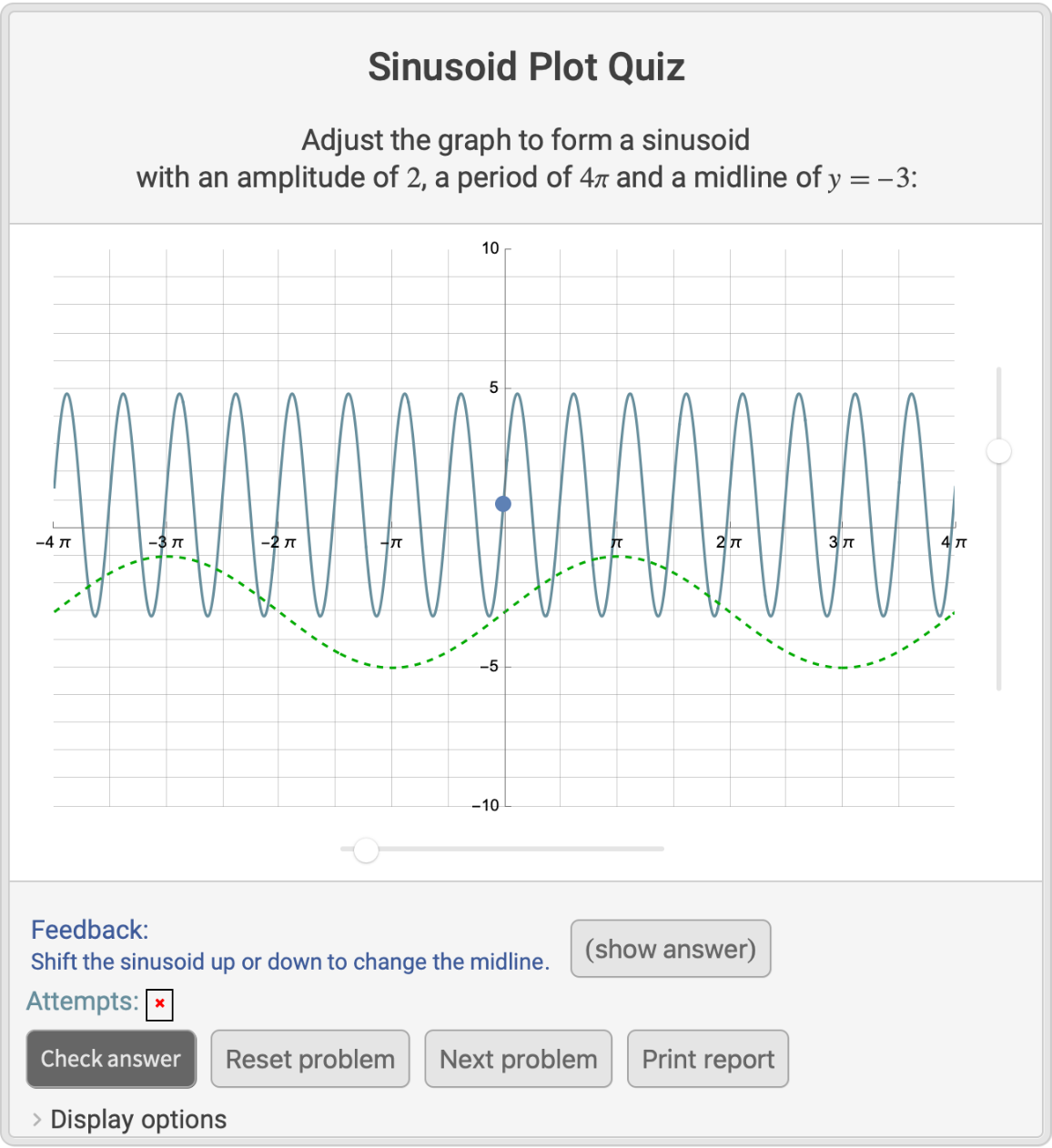Use the "Display options" menu to adjust grid lines, show the midline or key points of the sinusoid, retain the curve of the starting sinusoid, label the sliders or display a dynamically updating table showing the parameters of the sinusoid:

 In:=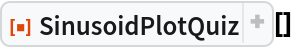Out=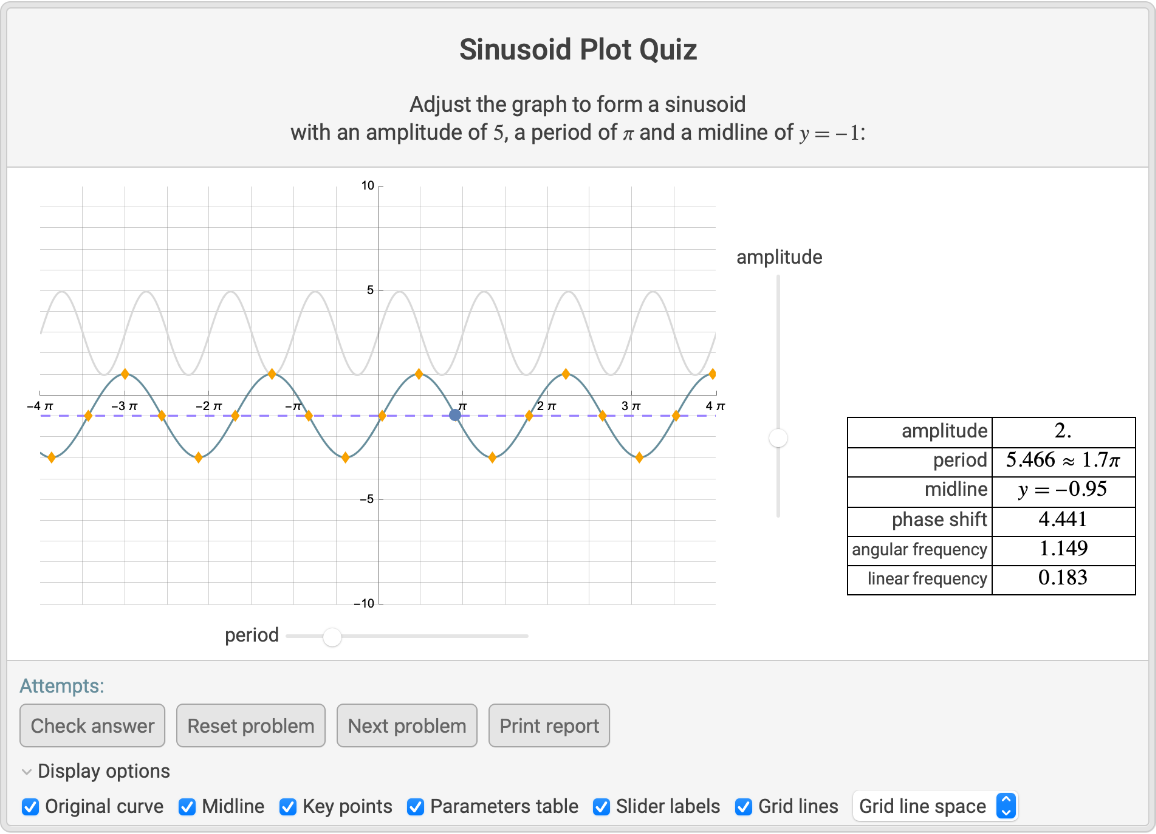Clicking the Print report button returns a description of the problems that have been attempted so far, all guesses made and whether the last guess on each problem was correct:

 In:=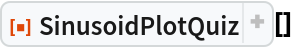Out=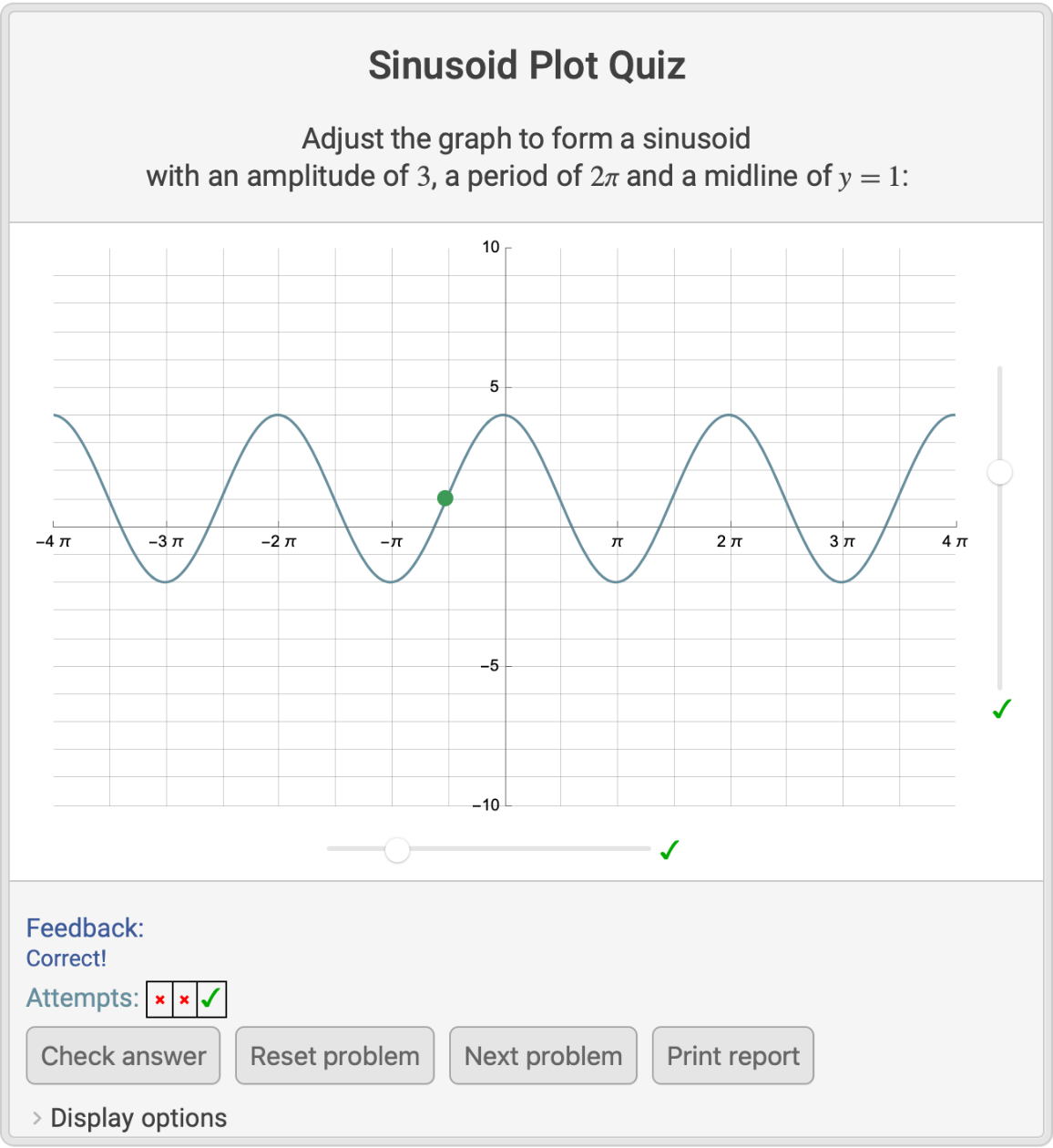Out=### Options (1)

Start every problem with the graph of an untranslated parent trigonometric function randomly chosen between Sin[x] and Cos[x]:

 In:=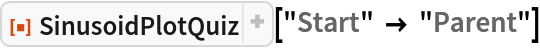Out=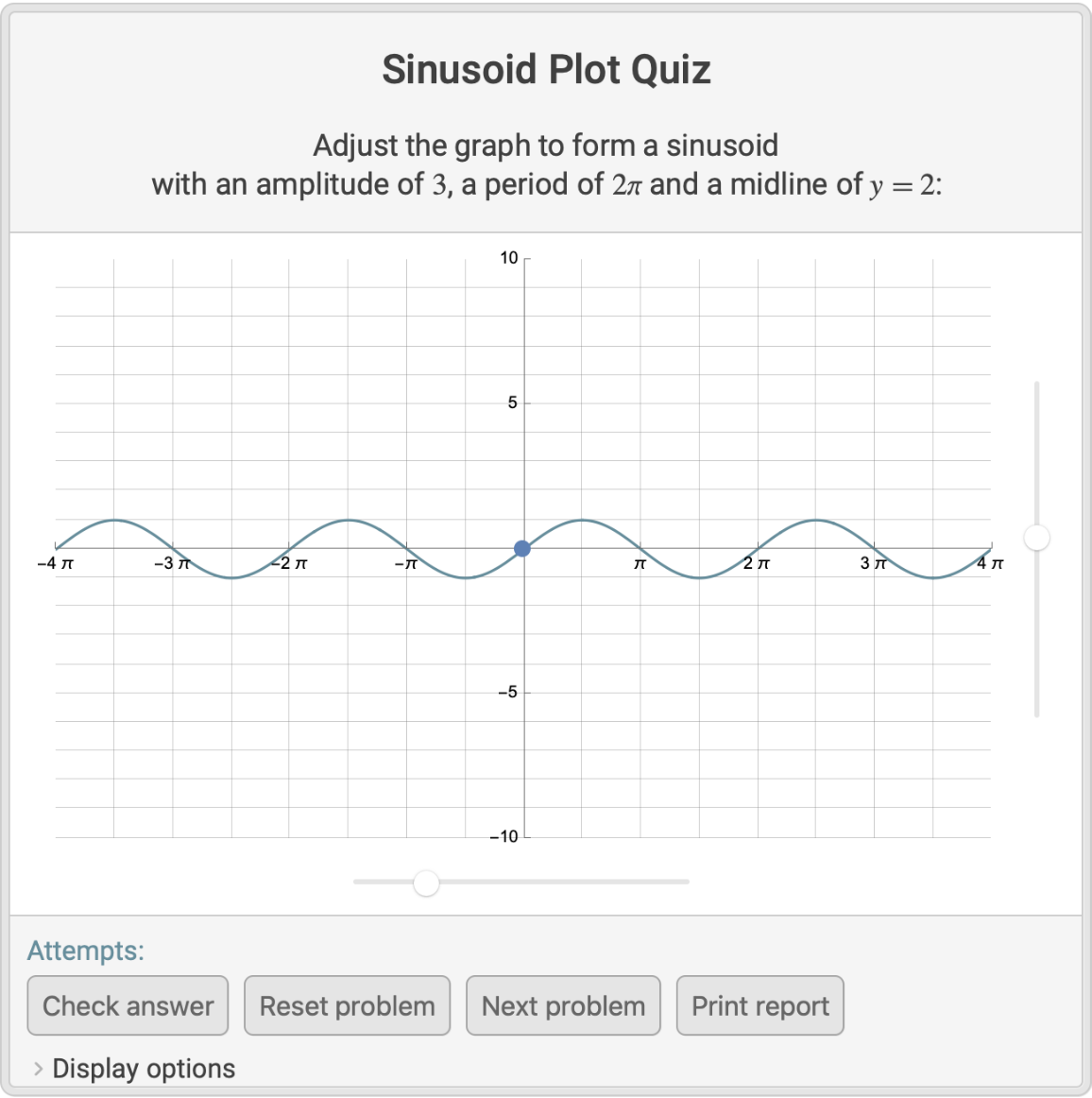## Publisher

Wolfram|Alpha Math Team

## Version History

• 4.0.0 – 23 March 2023
• 3.0.0 – 05 April 2022
• 2.0.0 – 10 March 2021
• 1.0.0 – 22 February 2021

## Author Notes

To view the full source code for SinusoidPlotQuiz, evaluate the following:

 In:=